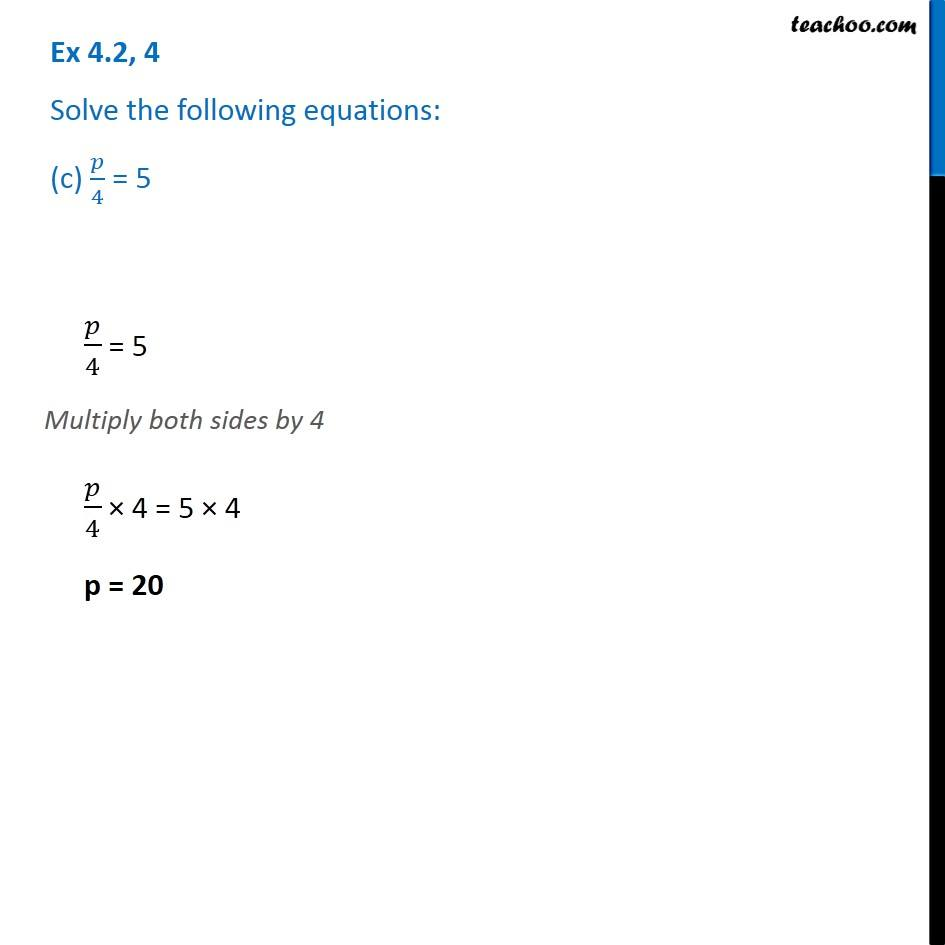1. Chapter 4 Class 7 Simple Equations
2. Serial order wise
3. Ex 4.2

Transcript

Ex 4.2, 4 Solve the following equations: (c) 𝑝/4 = 5𝑝/4 = 5 Multiply both sides by 4 𝑝/4 × 4 = 5 × 4 p = 20

Ex 4.2

Chapter 4 Class 7 Simple Equations
Serial order wise